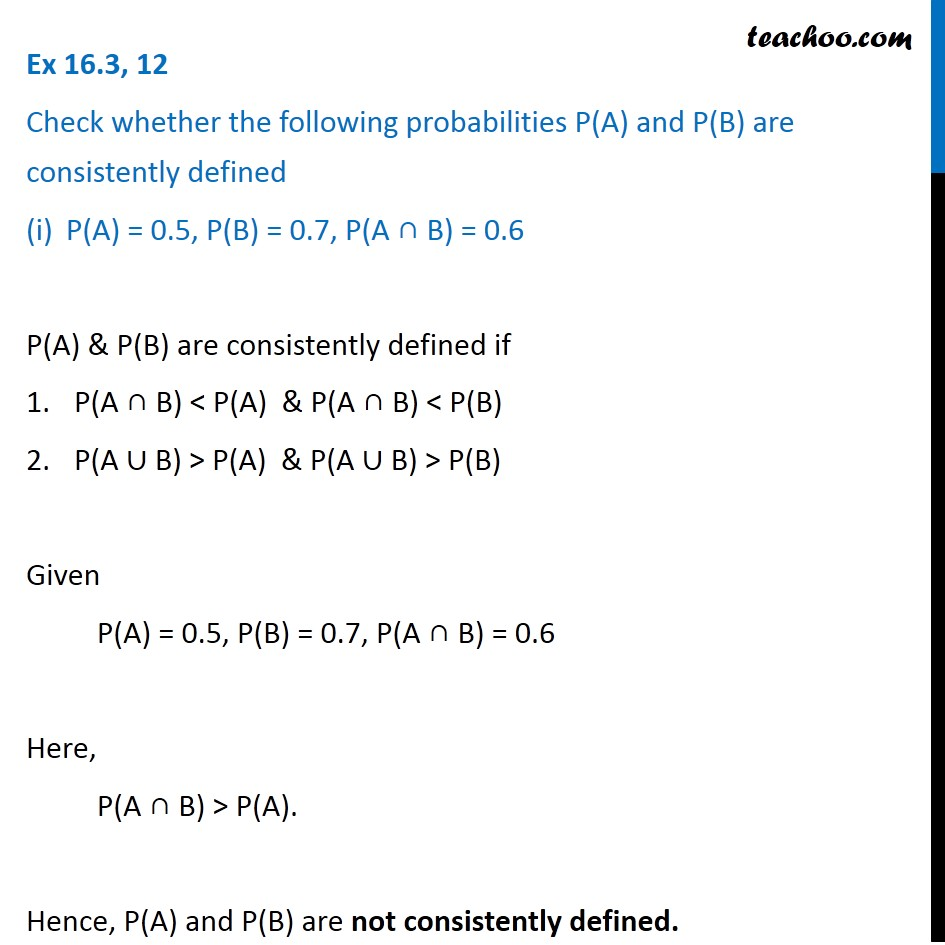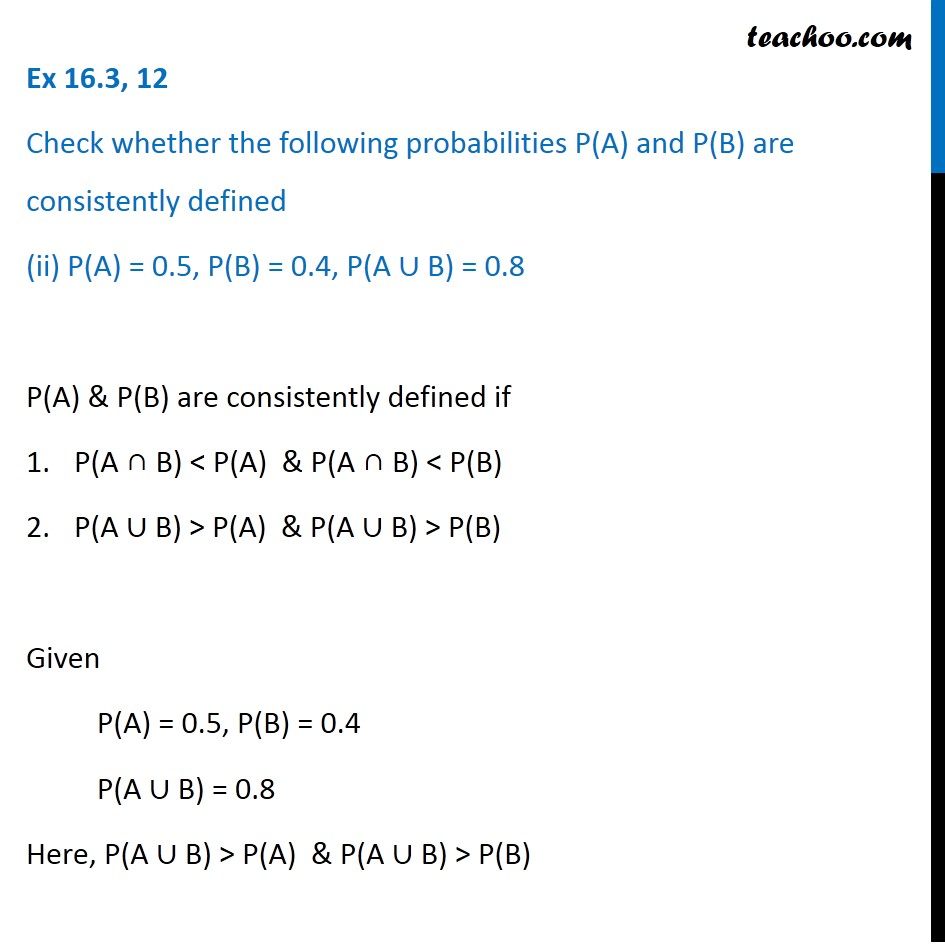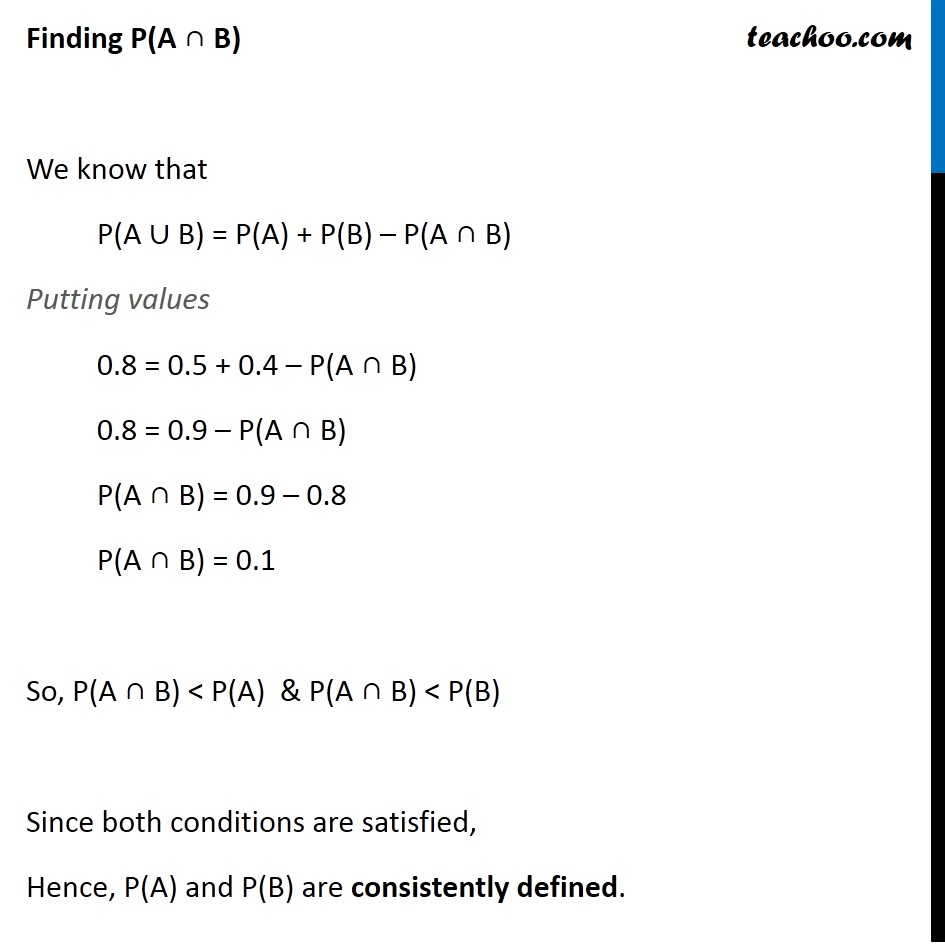Subscribe to our Youtube Channel - https://you.tube/teachoo

1. Chapter 16 Class 11 Probability
2. Serial order wise
3. Ex 16.3

Transcript

Ex 16.3, 12 Check whether the following probabilities P(A) and P(B) are consistently defined P(A) = 0.5, P(B) = 0.7, P(A ∩ B) = 0.6 P(A) & P(B) are consistently defined if P(A ∩ B) < P(A) & P(A ∩ B) < P(B) P(A ∪ B) > P(A) & P(A ∪ B) > P(B) Given P(A) = 0.5, P(B) = 0.7, P(A ∩ B) = 0.6 Here, P(A ∩ B) > P(A). Hence, P(A) and P(B) are not consistently defined. Note: Here we use combination as order of numbers is not important So, n(S) = 38760 To win a prize, there is only 1 case when six numbers match Let A be the event of winning lottery So, n(A) = 1 Probability of winning lottery P(A) = (𝑛(𝐴))/(𝑛(𝑆)) = 𝟏/𝟑𝟖𝟕𝟔𝟎 Ex 16.3, 12 Check whether the following probabilities P(A) and P(B) are consistently defined P(A) = 0.5, P(B) = 0.7, P(A ∩ B) = 0.6 P(A) & P(B) are consistently defined if P(A ∩ B) < P(A) & P(A ∩ B) < P(B) P(A ∪ B) > P(A) & P(A ∪ B) > P(B) Given P(A) = 0.5, P(B) = 0.7, P(A ∩ B) = 0.6 Here, P(A ∩ B) > P(A). Hence, P(A) and P(B) are not consistently defined. Ex 16.3, 12 Check whether the following probabilities P(A) and P(B) are consistently defined (ii) P(A) = 0.5, P(B) = 0.4, P(A ∪ B) = 0.8 P(A) & P(B) are consistently defined if P(A ∩ B) < P(A) & P(A ∩ B) < P(B) P(A ∪ B) > P(A) & P(A ∪ B) > P(B) Given P(A) = 0.5, P(B) = 0.4 P(A ∪ B) = 0.8 Here, P(A ∪ B) > P(A) & P(A ∪ B) > P(B) Finding P(A ∩ B) We know that P(A ∪ B) = P(A) + P(B) – P(A ∩ B) Putting values 0.8 = 0.5 + 0.4 – P(A ∩ B) 0.8 = 0.9 – P(A ∩ B) P(A ∩ B) = 0.9 – 0.8 P(A ∩ B) = 0.1 So, P(A ∩ B) < P(A) & P(A ∩ B) < P(B) Since both conditions are satisfied, Hence, P(A) and P(B) are consistently defined.

Ex 16.3